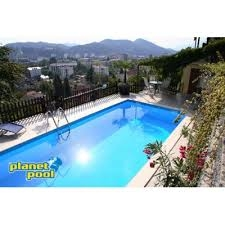# Temperature 7477

The pool with a length of l = 50 m and a width of s = 15 m has a depth of h1 = 1.2 m at the shallowest part of the wall. The depth then gradually increases to a depth of h2 = 1.5 m in the middle of the pool. = 4.5 m walls in the deepest part of the pool. Consider the specific heat capacity of water c = 4,200 J / (kg · ° C).

a) What is the volume of water in the pool?
b) How long does it take to fill the pool with two inlets, if each flows A = 5 liters / s?
c) How much GJ of heat is needed to heat the pool water by ∆t = 10 ° C?
d) When filling the pool for the first time, we require that the water has a temperature of t = 25 ° C.
How much hot water and how much cold water will we need if the temperature of cold water t1 = 10 ° C and hot water t2 = 60 ° C?

V =  1631.25 m3
t =  45.3125 h
E =  68.5125 GJ
a =  1141.875 m3
b =  489.375 m3

### Step-by-step explanation:Did you find an error or inaccuracy? Feel free to write us. Thank you!

Tips for related online calculators
Do you have a system of equations and looking for calculator system of linear equations?
Do you want to convert length units?
Do you know the volume and unit volume, and want to convert volume units?
Do you want to convert velocity (speed) units?

#### Grade of the word problem:

We encourage you to watch this tutorial video on this math problem: